## Stop and Wait Protocol-

Before you go through this article, make sure that you have gone through the previous article on Stop and Wait Protocol.

We have discussed-

• Stop and wait protocol is a simplest flow control protocol.
• Sender sends a data packet to the receiver and then waits for its acknowledgement.
• On receiving the acknowledgement, sender sends the next data packet.

Also Read- Stop and Wait ARQ

In this article, we will discuss practice problems based on stop and wait protocol.

## Problem-01:

If the bandwidth of the line is 1.5 Mbps, RTT is 45 msec and packet size is 1 KB, then find the link utilization in stop and wait.

## Solution-

Given-

• Bandwidth = 1.5 Mbps
• RTT = 45 msec
• Packet size = 1 KB

### Calculating Transmission Delay-

Transmission delay (Tt)

= Packet size / Bandwidth

= 1 KB / 1.5 Mbps

= (210 x 8 bits) / (1.5 x 106 bits per sec)

= 5.461 msec

### Calculating Propagation Delay-

Propagation delay (Tp)

= Round Trip Time / 2

= 45 msec / 2

= 22.5 msec

### Calculating Value Of ‘a’-

a = Tp / Tt

a = 22.5 msec / 5.461 msec

a = 4.12

= 1 / 1+2a

= 1 / (1 + 2 x 4.12)

= 1 / 9.24

= 0.108

= 10.8 %

## Problem-02:

A channel has a bit rate of 4 Kbps and one way propagation delay of 20 msec. The channel uses stop and wait protocol. The transmission time of the acknowledgement frame is negligible. To get a channel efficiency of at least 50%, the minimum frame size should be-

1. 80 bytes
2. 80 bits
3. 160 bytes
4. 160 bits

## Solution-

Given-

• Bandwidth = 4 Kbps
• Propagation delay (Tp) = 20 msec
• Efficiency >= 50%

Let the required frame size = L bits.

### Calculating Transmission Delay-

Transmission delay (Tt)

= Packet size / Bandwidth

= L bits / 4 Kbps

### Calculating Value Of ‘a’-

a = Tp / Tt

a = 20 msec / ( L bits / 4 Kbps)

a = (20 msec x 4 Kbps) / L bits

### Condition For Efficiency To Be At least 50%-

For efficiency to be at least 50%, we must have-

1 / 1+2a >= 1/2

a <= 1/2

Substituting the value of ‘a’, we get-

(20 msec x 4 Kbps) / L bits <= 1/2

L bits >= (20 msec x 4 Kbps) x 2

L bits >= (20 x 10-3 sec x 4 x 103 bits per sec) x 2

L bits >= 20 x 4 bits x 2

L >= 160

From here, frame size must be at least 160 bits.

Thus, Correct Option is (D).

## Problem-03:

What is the throughput achievable in stop and wait protocol by a maximum packet size of 1000 bytes and network span of 10 km.

Assume the speed of light in cable is 70% of the speed of light in vaccum.

## Solution-

We have-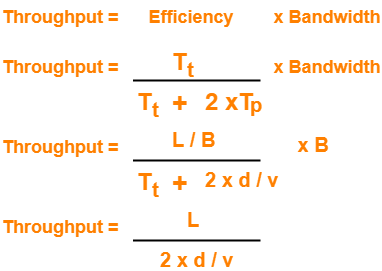• In the given question, we are not provided with the network’s bandwidth.
• So, in the above formula of throughput, we have ignored the term Tt from the denominator.
• Although it is incorrect, but we still ignore it for solving the question.

Now, Given-

• L = 1000 bytes
• d = 10 km = 104 m
• v = 70% of 3 x 108 m/sec = 2.1 x 108 m/sec

Substituting the values in the above relation, we get-

Throughput

= 1000 bytes / [ 2 x 104 m / (2.1 x 108 m/sec)]

= 1.05 x 107 bytes per sec

= 10.5 MBps

## Problem-04:

If the packet size is 1 KB and propagation time is 15 msec, the channel capacity is 109 b/sec, then find the transmission time and utilization of sender in stop and wait protocol.

## Solution-

Given-

• Packet size = 1 KB
• Propagation time (Tp) = 15 msec
• Channel capacity = Bandwidth (here) = 109 b/sec

### NOTE-

• Generally, channel capacity is the total number of bits which a channel can hold. So, its unit is bits.
• But here, channel capacity is actually given as bandwidth because its unit is b/sec.

### Calculating Transmission Delay-

Transmission delay (Tt)

= Packet size / Bandwidth

= 1 KB / 109 bits per sec

= 210 bits / 109 bits per sec

= 1.024 μsec

### Calculating Value Of ‘a’-

a = Tp / Tt

a = 15 msec / 1.024 μsec

a = 15000 μsec / 1.024 μsec

a = 14648.46

### Calculating Sender Utilization-

Sender Utilization or Efficiency (η)

= 1 / 1+2a

= 1 / (1 + 2 x 1468.46)

= 1 / 29297.92

= 0.0000341

= 0.00341 %

## Problem-05:

Consider a MAN with average source and destination 20 Km apart and one way delay of 100 μsec. At what data rate does the round trip delay equals the transmission delay for a 1 KB packet?

## Solution-

Given-

• Distance = 20 Km
• Propagation delay (Tp) = 100 μsec
• Packet size = 1 KB

We need to have-

Round Trip Time = Transmission delay

2 x Propagation delay = Transmission delay

Substituting the values in the above relation, we get-

2 x 100 μsec = 1 KB / Bandwidth

Bandwidth = 1 KB / 200 μsec

Bandwidth = (210 x 106 / 200 ) bytes per sec

Bandwidth = 5.12 MBps or 40.96 Mbps

## Problem-06:

Consider two hosts X and Y connected by a single direct link of rate 106 bits/sec. The distance between the two hosts is 10,000 km and the propagation speed along the link is 2 x 108 m/sec. Host X sends a file of 50,000 bytes as one large message to host Y continuously. Let the transmission and propagation delays be p milliseconds and q milliseconds respectively.

Then the value of p and q are-

1. p = 50 and q = 100
2. p = 50 and q = 400
3. p = 100 and q = 50
4. p = 400 and q = 50

## Solution-

Given-

• Bandwidth = 106 bits/sec
• Distance = 10,000 km
• Propagation speed = 2 x 108 m/sec
• Packet size = 50,000 bytes

### Calculating Transmission Delay-

Transmission delay (Tt)

= Packet size / Bandwidth

= 50000 bytes / 106 bits per sec

= (5 x 104 x 8 bits) / 106 bits per sec

= ( 4 x 105 bits ) / 106 bits per sec

= 0.4 sec

= 400 msec

### Calculating Propagation Delay-

Propagation delay (Tp)

= Distance / Propagation speed

= 10000 km / (2 x 108 m/sec)

= 107 m / (2 x 108 m/sec)

= 50 msec

Thus, Option (D) is correct.

## Problem-07:

The values of parameters for the stop and wait ARQ protocol are as given below-

• Bit rate of the transmission channel = 1 Mbps
• Propagation delay from sender to receiver = 0.75 ms
• Time to process a frame = 0.25 ms
• Number of bytes in the information frame = 1980
• Number of bytes in the acknowledge frame = 20
• Number of overhead bytes in the information frame = 20

Assume that there are no transmission errors. Then the transmission efficiency (in %) of the stop and wait ARQ protocol for the above parameters is ___________ . (correct to 2 decimal places)

## Solution-

Given-

• Bandwidth = 1 Mbps
• Propagation delay (Tp) = 0.75 ms
• Processing time (Tprocess) = 0.25 ms
• Data frame size = 1980 bytes
• Acknowledgement frame size = 20 bytes
• Overhead in data frame = 20 bytes

### Calculating Useful Time-

Useful data sent

= Transmission delay of useful data bytes sent

= Useful data bytes sent / Bandwidth

= (1980 bytes – 20 bytes) / 1 Mbps

= 1960 bytes / 1 Mbps

= (1960 x 8 bits) / (106 bits per sec)

= 15680 μsec

= 15.680 msec

### Calculating Total Time-

Total time

= Transmission delay of data frame + Propagation delay of data frame + Processing delay of data frame + Transmission delay of acknowledgement + Propagation delay of acknowledgement

= (1980 bytes / 1 Mbps) + 0.75 msec + 0.25 msec + (20 bytes / 1 Mbps) + 0.75 msec

= 15.840 msec + 0.75 msec + 0.25 msec + 0.160 msec + 0.75 msec

= 17.75 msec

### Calculating Efficiency-

Efficiency (η)

= Useful time / Total time

= 15.680 msec / 17.75 msec

= 0.8833

= 88.33%

## Problem-08:

A sender uses the stop and wait ARQ protocol for reliable transmission of frames. Frames are of size  1000 bytes and the transmission rate at the sender is 80 Kbps. Size of an acknowledgement is 100 bytes and the transmission rate at the receiver is 8 Kbps. The one way propagation delay is 100 msec.

Assuming no frame is lost, the sender throughput is __________ bytes/sec.

## Solution-

Given-

• Frame size = 1000 bytes
• Sender bandwidth = 80 Kbps
• Acknowledgement size = 100 bytes
• Receiver bandwidth = 8 Kbps
• Propagation delay (Tp) = 100 msec

### Calculating Transmission Delay Of Data Frame-

Transmission delay (Tt)

= Frame size / Sender bandwidth

= 1000 bytes / 80 Kbps

= (1000 x 8 bits) / (80 x 103 bits per sec)

= 0.1 sec

= 100 msec

### Calculating Transmission Delay Of Acknowledgement-

Transmission delay (Tt)

= Acknowledgement size / Receiver bandwidth

= 100 bytes / 8 Kbps

= (100 x 8 bits) / (8 x 103 bits per sec)

= 100 msec

### Calculating Useful Time-

Useful Time

= Transmission delay of data frame

= 100 msec

### Calculating Total Time-

Total Time

= Transmission delay of data frame + Propagation delay of data frame + Transmission delay of acknowledgement + Propagation delay of acknowledgement

= 100 msec + 100 msec + 100 msec + 100 msec

= 400 msec

### Calculating Efficiency-

Efficiency (η)

= Useful time / Total time

= 100 msec / 400 msec

= 1 / 4

= 25%

### Calculating Sender Throughput-

Sender throughput

= Efficiency (η) x Sender bandwidth

= 0.25 x 80 Kbps

= 20 Kbps

= (20 x 1000 / 8) bytes per sec

= 2500 bytes/sec

## Problem-09:

Using stop and wait protocol, sender wants to transmit 10 data packets to the receiver. Out of these 10 data packets, every 4th data packet is lost. How many packets sender will have to send in total?

## Solution-

Draw a time line diagram and analyze.

The packets will be sent as-

1, 2, 3, 4, 4, 5, 6, 7, 7, 8, 9, 10, 10

The lost packets are- 4, 7 and 10.

Thus, sender will have to send 13 data packets in total.

Next Article- Sliding Window Protocol

Get more notes and other study material of Computer Networks.

Watch video lectures by visiting our YouTube channel LearnVidFun.

## Stop and Wait Protocol-

Before you go through this article, make sure that you have gone through the previous article on Stop and Wait Protocol.

In stop and wait protocol,

• Sender sends one data packet and then waits for its acknowledgement.
• Sender sends the next packet only after it receives the acknowledgement for the previous packet.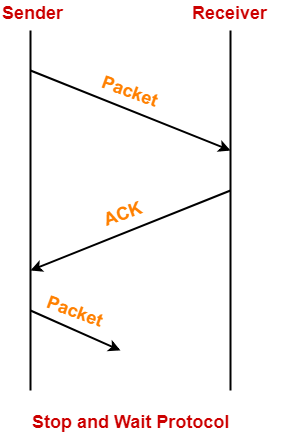The main problem faced by the Stop and Wait protocol is the occurrence of deadlock due to-

1. Loss of data packet
2. Loss of acknowledgement

## Stop and Wait ARQ-

 Stop and Wait ARQ is an improved and modified version of Stop and Wait protocol.

Stop and Wait ARQ assumes-

• The communication channel is noisy.
• Errors may get introduced in the data during the transmission.

## Working-

• Stop and wait ARQ works similar to stop and wait protocol.
• It provides a solution to all the limitations of stop and wait protocol.
• Stop and wait ARQ includes the following three extra elements.Thus, we can say-

 Stop and Wait ARQ= Stop and Wait Protocol + Time Out Timer + Sequence Numbers for Data Packets and Acknowledgements

## NOTE

For any sliding window protocol to work without any problem,

the following condition must be satisfied-

Available Sequence Numbers >= Sender Window Size + Receiver Window Size

Stop and wait ARQ is a one bit sliding window protocol where-

• Sender window size = 1
• Receiver window size = 1

Thus, in stop and wait ARQ,

Minimum number of sequence numbers required

= Sender Window Size + Receiver Window Size

= 1 + 1

= 2

Thus,

• Minimum number of sequence numbers required in Stop and Wait ARQ = 2.
• The two sequence numbers used are 0 and 1.

## 1. Problem of Lost Data Packet-

• Time out timer helps to solve the problem of lost data packet.
• After sending a data packet  to the receiver, sender starts the time out timer.
• If the data packet gets acknowledged before the timer expires, sender stops the time out timer.
• If the timer goes off before receiving the acknowledgement, sender retransmits the same data packet.
• After retransmission, sender resets the timer.
• This prevents the occurrence of deadlock.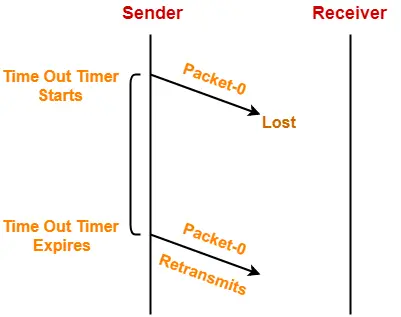## 2. Problem of Lost Acknowledgement-

• Sequence number on data packets help to solve the problem of delayed acknowledgement.
• Consider the acknowledgement sent by the receiver gets lost.
• Then, sender retransmits the same data packet after its timer goes off.
• This prevents the occurrence of deadlock.
• The sequence number on the data packet helps the receiver to identify the duplicate data packet.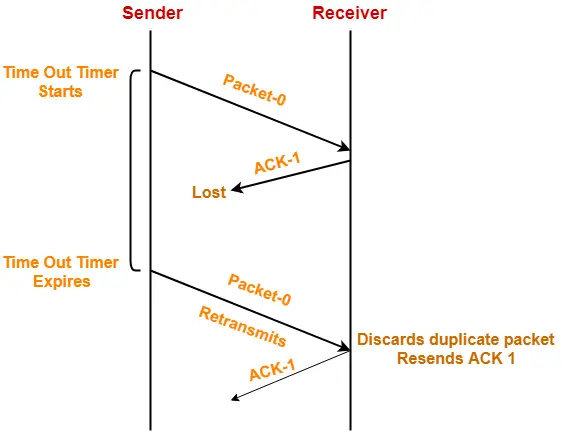## Role of Sequence Number on Data Packets

Consider the above example-

### Step-01:

• Sender sends a data packet with sequence number-0 to the receiver.

### Step-02:

• Receiver now expects data packet with sequence number-1.
• Receiver sends the acknowledgement ACK-1.

### Step-03:

• Acknowledgement ACK-1 sent by the receiver gets lost on the way.

### Step-04:

• Sender receives no acknowledgement and time out occurs.
• Sender retransmits the same data packet with sequence number-0.
• This will be a duplicate packet for the receiver.

### Step-05:

• Receiver receives the data packet and discovers it is the duplicate packet.
• It expects the data packet with sequence number-1 but receiving the data packet with sequence number-0.
• It discards the duplicate data packet and re-sends acknowledgement ACK-1.
• ACK-1 requests the sender to send a data packet with sequence number-1.
• This avoids the inconsistency of data.

### Conclusion-

• Had the sequence numbers not been allotted to the data packets, receiver would have accepted the duplicate data packet thinking of it as the new data packet.
• This is how sequence numbers allotted to the data packets prove to be useful for identifying the duplicate data packets and discarding them.

## 3. Problem of Delayed Acknowledgement-

• Sequence number on acknowledgements help to solve the problem of delayed acknowledgement.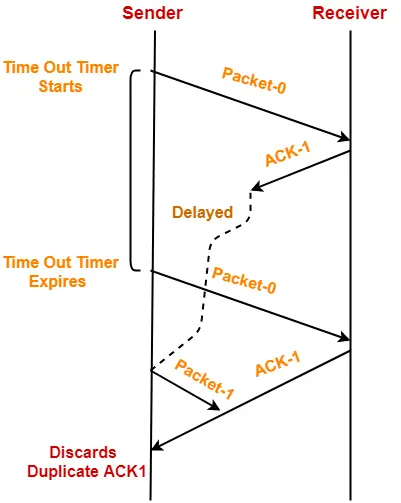## Role of Sequence Number on Acknowledgements

Consider the above example-

### Step-01:

• Sender sends a data packet with sequence number-0 to the receiver.

### Step-02:

• Receiver now expects data packet with sequence number-1.
• Receiver sends the acknowledgement ACK-1.

### Step-03:

• Acknowledgement ACK-1 sent by the receiver gets delayed in reaching the sender.

### Step-04:

• Sender receives no acknowledgement and time out occurs.
• Sender retransmits the same data packet with sequence number-0.
• This will be a duplicate packet for the receiver.

### Step-05:

• Receiver receives the data packet and discovers it is the duplicate packet.
• It expects the data packet with sequence number-1 but receiving the data packet with sequence number-0.
• It discards the duplicate data packet and re-sends acknowledgement ACK-1.
• ACK-1 requests the sender to send a data packet with sequence number-1.

### Step-06:

• Two acknowledgements ACK1 reaches the sender.
• When first acknowledgement ACK1 reaches the sender, sender sends the next data packet with sequence number 1.
• When second acknowledgement ACK1 reaches the sender, sender rejects the duplicate acknowledgement.
• This is because it has already sent the data packet with sequence number-1 and now sender expects the acknowledgement with sequence number 0 from the receiver.

### Conclusion-

• Had the sequence numbers not been allotted to the acknowledgements, sender would have accepted the duplicate acknowledgement thinking of it as the new acknowledgement for the latest data packet sent by it.
• This is how sequence numbers allotted to the acknowledgements prove to be useful for identifying duplicate acknowledgements and discarding them.

## 4. Problem of Damaged Packet-

• If receiver receives a corrupted data packet from the sender, it sends a negative acknowledgement (NAK) to the sender.
• NAK requests the sender to send the data packet again.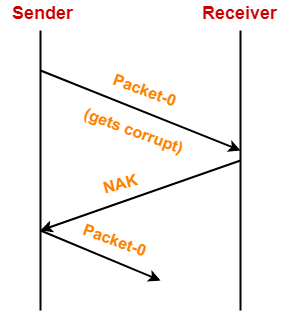## Stop and Wait Protocol Vs Stop and Wait ARQ-

The following comparison table states the differences between the two protocols-

 Stop and Wait Protocol Stop and Wait ARQ It assumes that the communication channel is perfect and noise free. It assumes that the communication channel is imperfect and noisy. Data packet sent by the sender can never get corrupt. Data packet sent by the sender may get corrupt. There is no concept of negative acknowledgements. A negative acknowledgement is sent by the receiver if the data packet is found to be corrupt. There is no concept of time out timer. Sender starts the time out timer after sending the data packet. There is no concept of sequence numbers. Data packets and acknowledgements are numbered using sequence numbers.

## Limitation of Stop and Wait ARQ-

 The major limitation of Stop and Wait ARQ is its very less efficiency.

To increase the efficiency, protocols like Go back N and Selective Repeat are used.

## Explanation-

In stop and wait ARQ,

• Sender window size is 1.
• This allows the sender to keep only one frame unacknowledged.
• So, sender sends one frame and then waits until the sent frame gets acknowledged.
• After receiving the acknowledgement from the receiver, sender sends the next frame.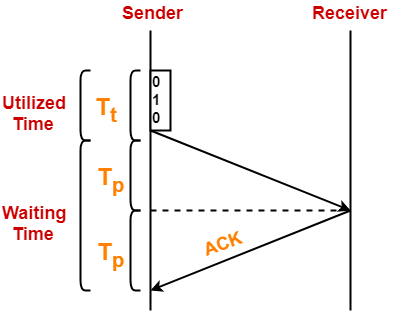Here,

• Sender uses Tt time for transmitting the packet over the link.
• Then, sender waits for 2 x Tp time.
• After 2 x Tp time, sender receives the acknowledgement for the sent frame from the receiver.
• Then, sender sends the next frame.
• This 2 x Tp waiting time is the actual cause of less efficiency.

## Efficiency Improvement-

• The efficiency of stop and wait ARQ can be improved by increasing the window size.
• This allows the sender to keep more than one unacknowledged frame in its window.
• Thus, sender can send frames in the waiting time too.

This gives rise to the concept of sliding window protocols.

To gain better understanding about Stop and Wait ARQ,

Watch this Video Lecture

Next Article- Practice Problems On Stop and Wait Protocol

Get more notes and other study material of Computer Networks.

Watch video lectures by visiting our YouTube channel LearnVidFun.